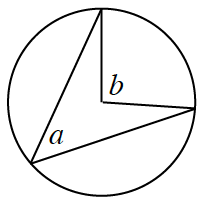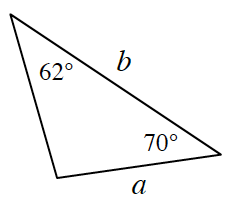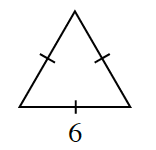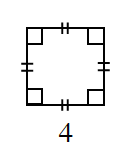### Home > INT2 > Chapter 12 > Lesson 12.2.1 > Problem12-61

12-61.

For each geometric relationship below, determine whether $a$ or $b$ is larger, or if they are equal. Assume that the diagrams are not drawn to scale. If there is not enough information, explain what information is missing.

1.1.1.area of the triangle is $a$ area of the square is $b$

Remember that inscribed angles measure half of central angles.